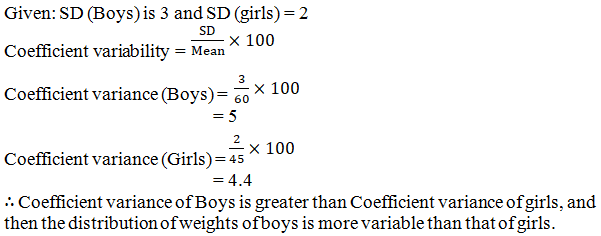# RD Sharma Solutions for Class 11 Chapter 32 - Statistics Exercise 32.7

In this section, we shall see how we can use various measures of dispersion to compare two or more series. The solutions prepared by our expert faculty team help students in their exam preparation to secure full marks in Maths. The Solutions given in this page contains detailed step-by-step explanations to all the problems that come under this chapter. These solutions will help you in gaining knowledge and strong command over the subject. If you wish to secure an excellent score, solving RD Sharma Class 11 Maths Solutions is a must. Here is the RD Sharma Solutions for Class 11 Chapter 32 where solutions are solved in detail. Students can download the pdf of this chapter, in their respective links.

## Download the pdf of RD Sharma Solutions for Class 11 Maths Exercise 32.7 Chapter 32 – Statistics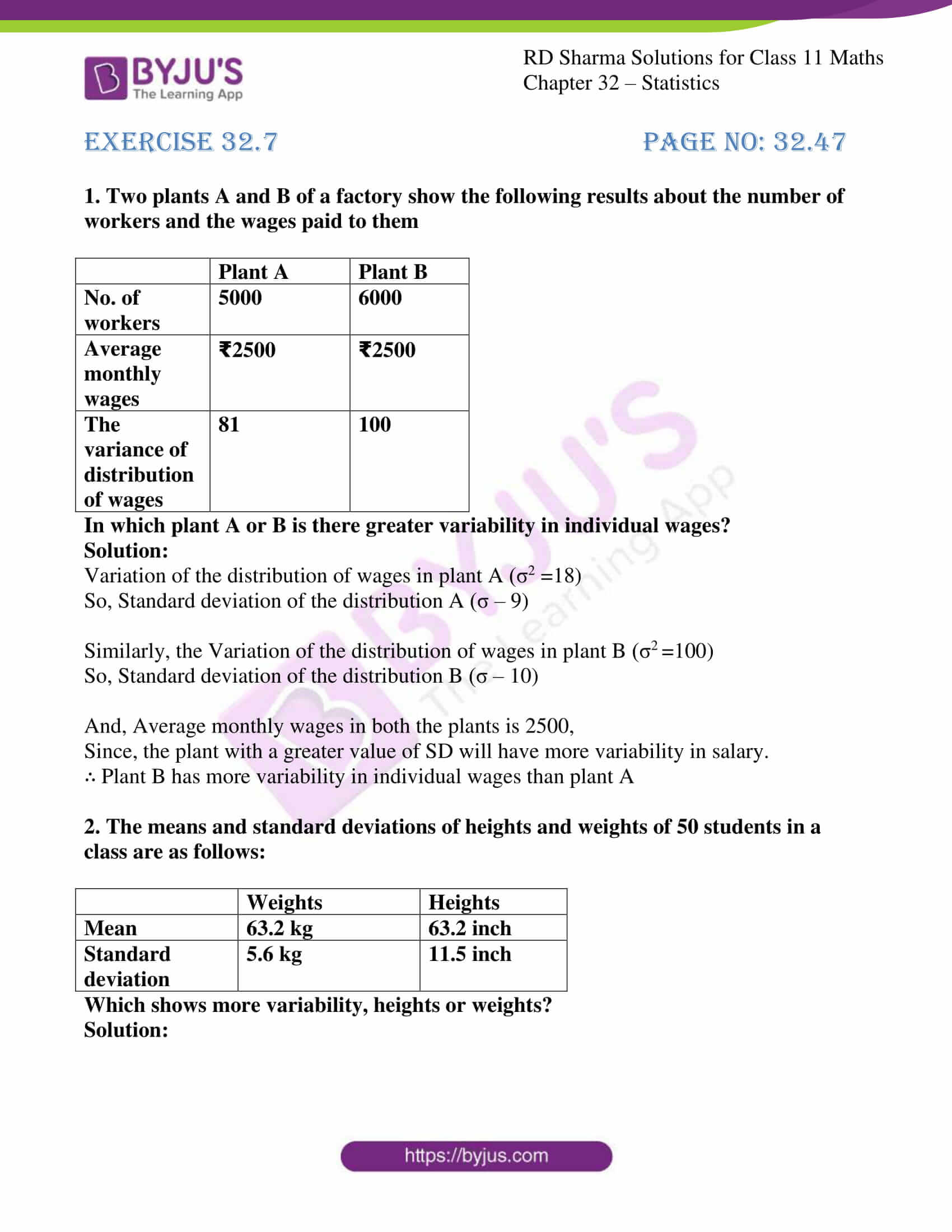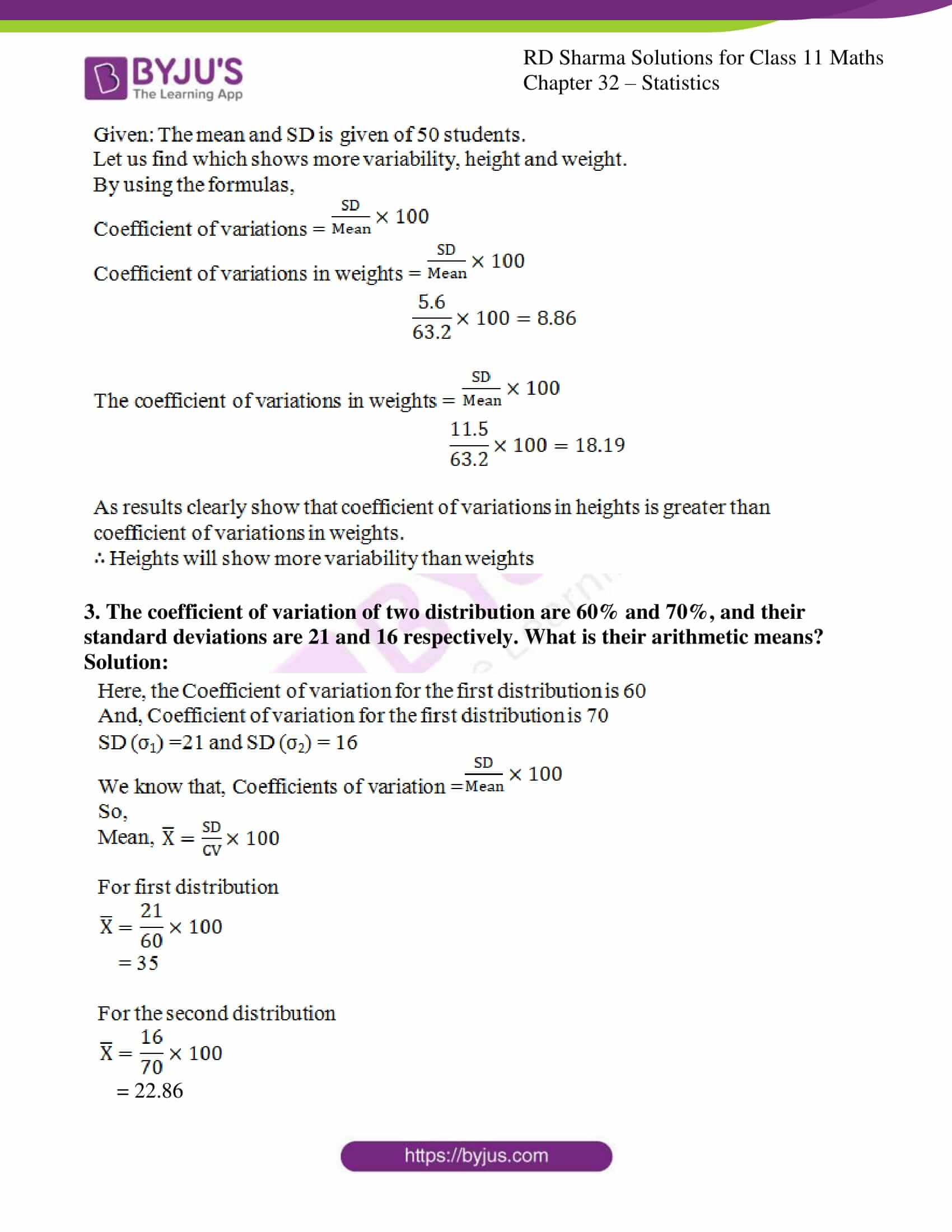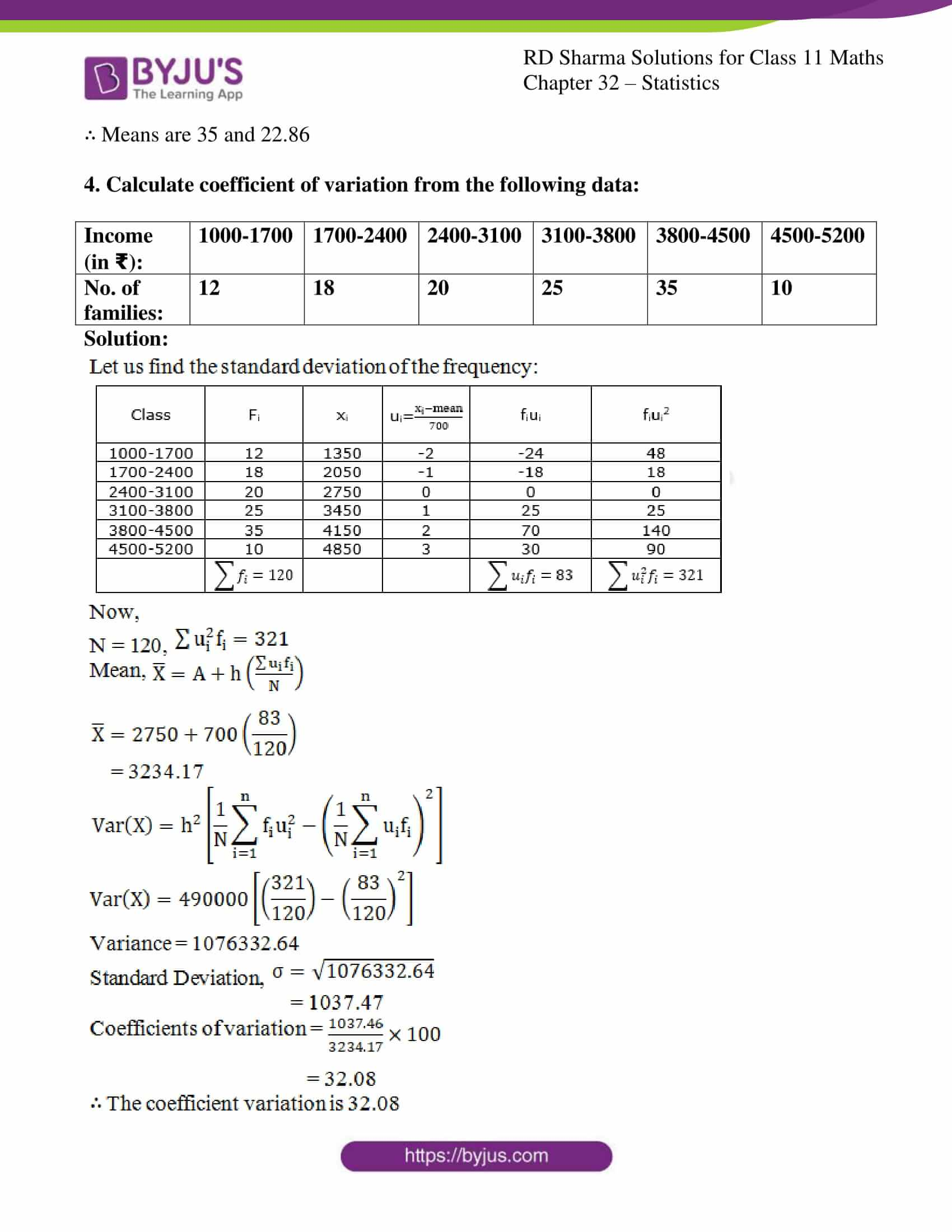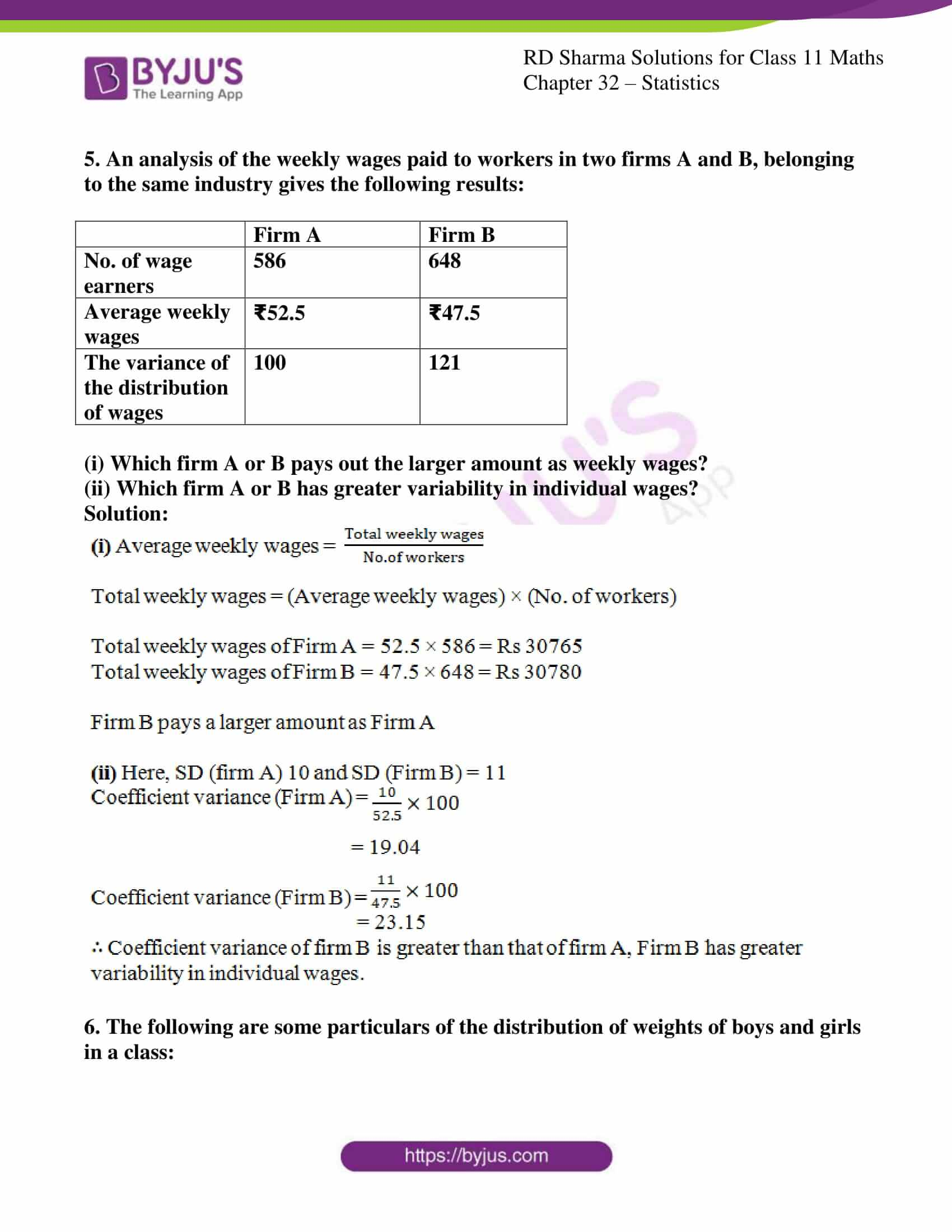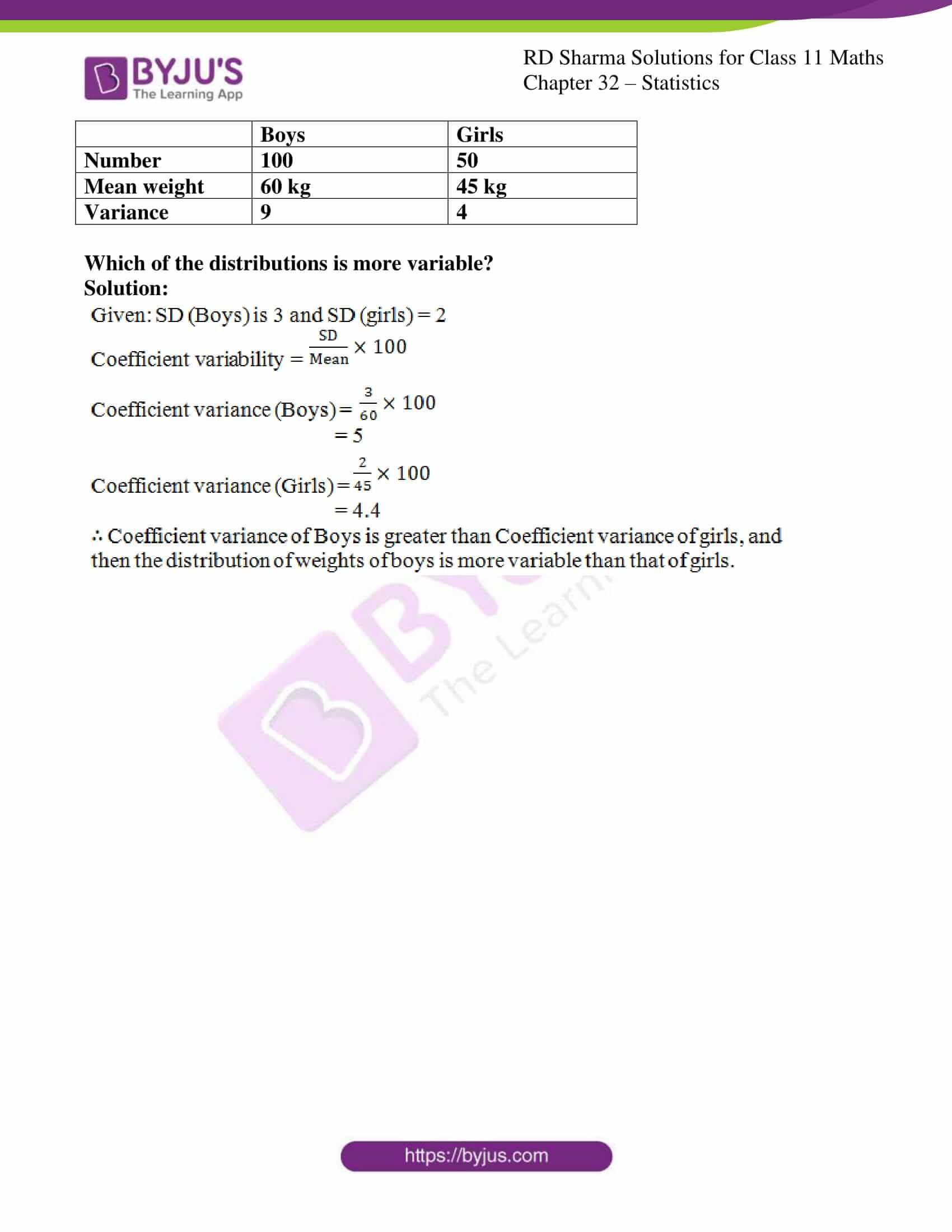### Access answers to RD Sharma Solutions for Class 11 Maths Exercise 32.7 Chapter 32 – Statistics

#### EXERCISE 32.7 PAGE NO: 32.47

1. Two plants A and B of a factory show the following results about the number of workers and the wages paid to them

 Plant A Plant B No. of workers 5000 6000 Average monthly wages ₹2500 ₹2500 The variance of distribution of wages 81 100

In which plant A or B is there greater variability in individual wages?

Solution:

Variation of the distribution of wages in plant A (σ2 =18)

So, Standard deviation of the distribution A (σ – 9)

Similarly, the Variation of the distribution of wages in plant B (σ2 =100)

So, Standard deviation of the distribution B (σ – 10)

And, Average monthly wages in both the plants is 2500,

Since, the plant with a greater value of SD will have more variability in salary.

∴ Plant B has more variability in individual wages than plant A

2. The means and standard deviations of heights and weights of 50 students in a class are as follows:

 Weights Heights Mean 63.2 kg 63.2 inch Standard deviation 5.6 kg 11.5 inch

Which shows more variability, heights or weights?

Solution: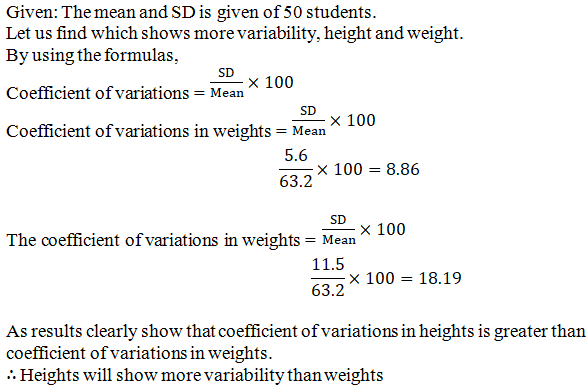3. The coefficient of variation of two distribution are 60% and 70%, and their standard deviations are 21 and 16 respectively. What is their arithmetic means?

Solution: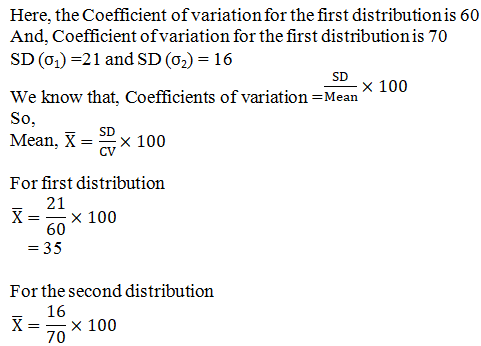= 22.86

∴ Means are 35 and 22.86

4. Calculate coefficient of variation from the following data:

 Income (in ₹): 1000-1700 1700-2400 2400-3100 3100-3800 3800-4500 4500-5200 No. of families: 12 18 20 25 35 10

Solution: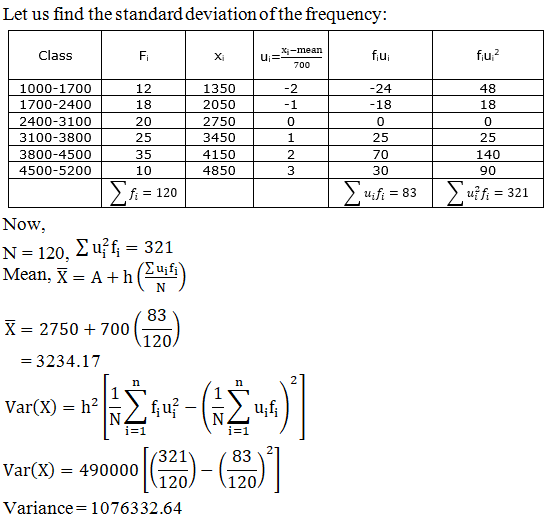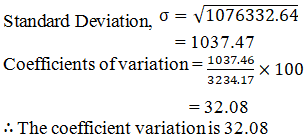5. An analysis of the weekly wages paid to workers in two firms A and B, belonging to the same industry gives the following results:

 Firm A Firm B No. of wage earners 586 648 Average weekly wages ₹52.5 ₹47.5 The variance of the distribution of wages 100 121

(i) Which firm A or B pays out the larger amount as weekly wages?
(ii) Which firm A or B has greater variability in individual wages?

Solution: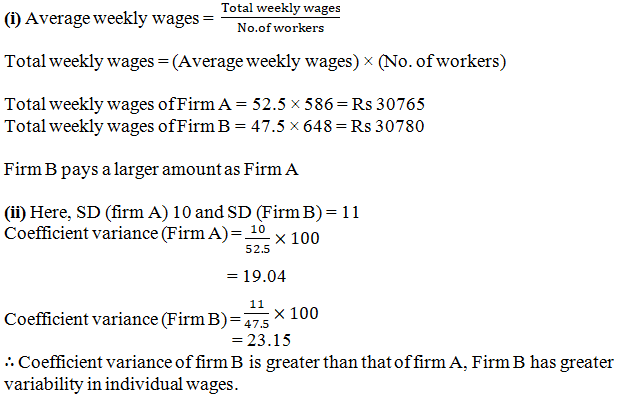6. The following are some particulars of the distribution of weights of boys and girls in a class:

 Boys Girls Number 100 50 Mean weight 60 kg 45 kg Variance 9 4

Which of the distributions is more variable?

Solution: